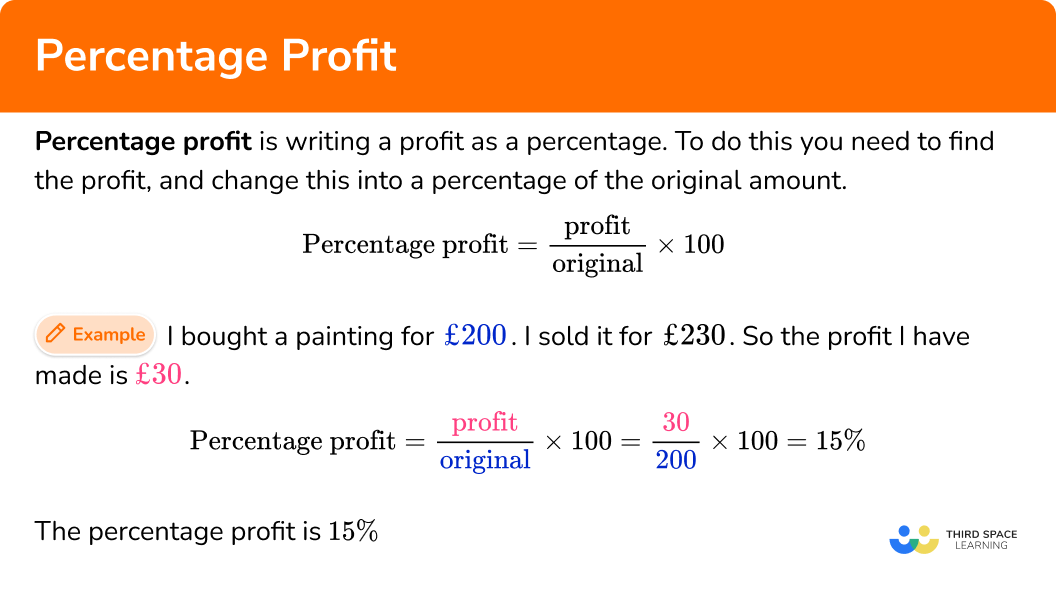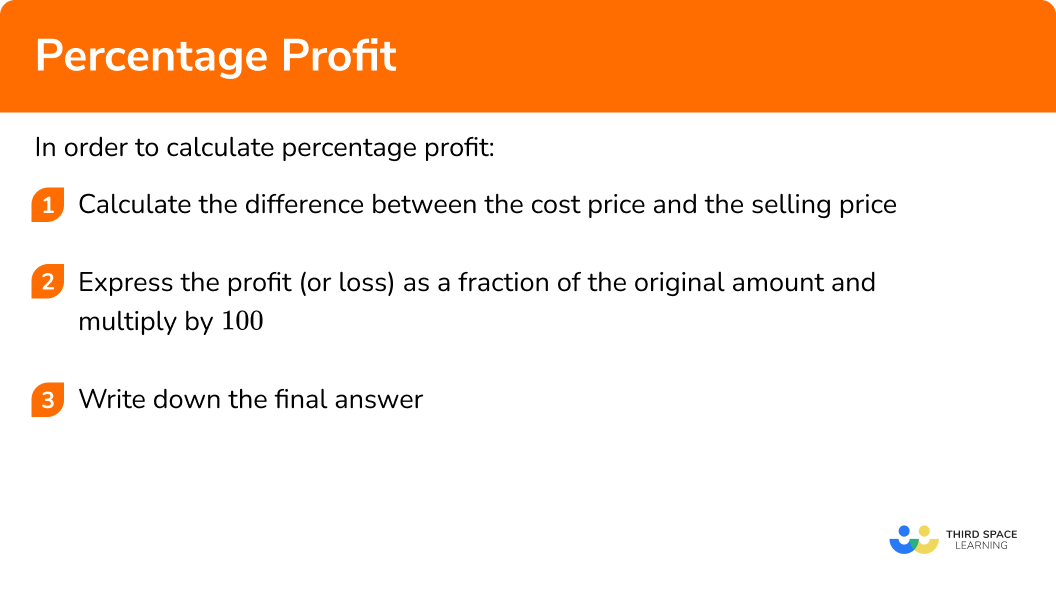GCSE Maths Number FDP Percentages

Percentage Profit

# Percentage Profit

Here we will learn about percentage profit, including what percentage profit is and how to solve problems involving percentage profit.

There are also percentage profit worksheets based on Edexcel, AQA and OCR exam questions, along with further guidance on where to go next if you’re still stuck.

## What is percentage profit?

Percentage profit is writing a profit as a percentage. This makes it easier to compare with other profits.

To do this you need to find the profit and change this into a percentage of the original amount (the cost of the item at the start). Calculating profit is done by finding the difference between the cost price and the selling price.

You can use this formula to calculate the profit percentage,

\text{Percentage profit}=\cfrac{\text{profit}}{\text{original}}\times 100 .

For example, I bought a painting for £200. I sold it for £230. So the profit I have made is £30 as this is the difference between the cost price and the selling price.

\text{Percentage profit}=\cfrac{\text{profit}}{\text{original}}\times 100=\cfrac{30}{200}\times 100=15\%

The percentage profit is 15\%.

The amount of money the item is bought for at the start can be called the cost price (or cost of goods sold).

The amount of money the item is sold for later in the question can be called the sale price or the selling price.

This can be extended into calculating percentage loss. This is when the value has gone down over time (known as depreciation).

\text{Percentage loss}=\cfrac{\text{loss}}{\text{original}}\times 100

This skill is very useful for business owners.

### What is percentage profit?## How to calculate percentage profit

In order to calculate percentage profit:

1. Calculate the difference between the cost price and the selling price.
2. Express the profit (or loss) as a fraction of the original amount and multiply by \bf{100} .
3. Write down the final answer.

### Explain how to calculate percentage profit## Percentage profit examples

### Example 1: calculating percentage profit

Ron bought an antique train set for £130.

He sold it for £215.

Calculate the percentage profit Ron made.

1. Calculate the difference between the cost price and the selling price.

The difference is,

215-130=85.

2Express the profit (or loss) as a fraction of the original amount and multiply by \bf{100} .

You can find the percentage profit by making a fraction and multiplying by 100.

\text{Percentage profit}=\cfrac{\text{profit}}{\text{original}}\times 100=\cfrac{85}{130}\times 100=65.384…

The percentage profit Ron made is \bf{65\%} to the nearest percent.

### Example 2: calculating percentage profit

Ellen buys a house for £210 \ 000. She sells the house for £226 \ 500.

Calculate the percentage profit Ellen makes. Give your answer to 1 decimal place.

The difference is,

226 \ 500-210 \ 000=16 \ 500.

You can find the percentage profit by making a fraction and multiplying by 100.

\text{Percentage profit}=\cfrac{\text{profit}}{\text{original}}\times 100=\cfrac{16 \ 500}{210 \ 000}\times 100=7.857…

The percentage profit Ellen makes is \bf{7.9\%} to 1 decimal place.

### Example 3: calculating percentage loss

Diane bought an antique vase for £95.

She sold it for £78.

Calculate the percentage loss Diane made.

The difference is,

95-78=17.

You can find the percentage loss by making a fraction and multiplying by 100.

\text{Percentage loss}=\cfrac{\text{loss}}{\text{original}}\times 100=\cfrac{17}{95}\times 100=17.894…

The percentage loss Diane made is \bf{17.9\%} to 3 significant figures.

### Example 4: calculating percentage loss

Rahul bought a van for £16 \ 500.

He sold it for £9 \ 350.

Calculate the percentage loss Rahul made.

The difference is,

16 \ 500-9 \ 350=7 \ 150.

You can find the percentage loss by making a fraction and multiplying by 100.

\text{Percentage loss}=\cfrac{\text{loss}}{\text{original}}\times 100=\cfrac{7 \ 150}{16 \ 500}\times 100=43.333…

The percentage loss Rahul makes is \bf{43.3\%} to 3 significant figures.

### Example 5: problem solving

Katie buys a pack of 12 bottles of water for £3.50.

She sells the water at 80p per bottle.

Work out Katie’s percentage profit.

First you need to calculate the total selling price (or total sales).

12\times 80=960

The total selling price is £9.60

The difference is,

£9.60-£3.50=£6.10 .

You can find the percentage profit by making a fraction and multiplying by 100.

\text{Percentage profit}=\cfrac{\text{profit}}{\text{original}}\times 100=\cfrac{6.10}{3.50}\times 100=174.285…

The percentage profit Katie makes is \bf{174\%}.

### Example 6: problem solving

Ranjeev buys 20 jackets at £25 each.

He sells 15\% of them for £50 each and half of them for £35. He sells the rest for £12 each.

Work out Ranjeev’s percentage profit or loss.

State clearly if Ranjeev makes a profit or a loss.

First you need to calculate the total cost price (or cost of goods sold).

20\times \pounds25=\pounds500

Then you need to work out the total selling price (or total sales).

15\% of 20 is 3 jackets, so he sells 3 jackets at £50. He also sells 10 jackets at £35 and 7 jackets at £12.

The total selling price is,

(3\times \pounds50) + (10\times \pounds35) + (7\times \pounds12)=\pounds584.

Ranjeev sells the jackets for more than he bought them for, so he has made a profit.

The difference is,

584-500=84 .

You can find the percentage profit by making a fraction and multiplying by 100.

\text{Percentage profit}=\cfrac{\text{profit}}{\text{original}}\times 100=\cfrac{84}{500}\times 100=16.8

Ranjeev makes a profit and the percentage profit is \bf{16.8\%}.

### Common misconceptions

• Always use the original cost price as the denominator

The original cost price is always used as the denominator in the percentage formula for profit.

• Be consistent with pounds and pence

Sometimes questions may give one amount of money in pence (p), and another amount of money in pounds (£). When you calculate the percentage profit (or loss) make sure you use the same type of monetary units in your fraction. Whichever you use will give the same percentage profit.

• Percentage profits can be more than \bf{100}

When calculating percentages for profits, they can be greater than 100\%. For example, if I buy a bottle of water for £0.50 and I sell it for £2, I made £1.50 profit which is 300\% profit. I sold the bottle for 4 times more than I originally bought it for.

Reverse percentages is part of our series of lessons to support revision on percentages. You may find it helpful to start with the main percentages lesson for a summary of what to expect, or use the step by step guides below for further detail on individual topics. Other lessons in this series include:

### Practice percentage profit questions

1. A trailer is bought for £50 and is sold for £60. Find the percentage profit.

20\%2\%16.7\%17\%The profit is £10.

\text{Percentage profit}=\cfrac{\text{profit}}{\text{original}}\times 100=\cfrac{10}{50}\times 100=20

The percentage profit is 20\%.

2. A flat is bought for £120 \ 000 and is sold for £145 \ 000. Find the percentage profit. Give your answer to the nearest percent.

25\%17\%28\%21\%The profit is £25 \ 000.

\text{Percentage profit}=\cfrac{\text{profit}}{\text{original}}\times 100=\cfrac{10}{50}\times 100=20.8333…

The percentage profit is 21\% (to the nearest percent).

3. A motorbike is bought for £995 and is sold for £850. Find the percentage loss. Give your answer to the nearest percent.

14\%15\%16\%17\%The loss is £145.

\text{Percentage profit}=\cfrac{\text{profit}}{\text{original}}\times 100=\cfrac{145}{995}\times 100=14.572…

The percentage loss is 15\% (to the nearest percent).

4. A flat is bought for £120 \ 000 and is sold for £106 \ 500. Find the percentage loss. Give your answer to the nearest percent.

11\%12\%13\%10\%The loss is £13500.

\text{Percentage profit}=\cfrac{\text{profit}}{\text{original}}\times 100=\cfrac{13500}{120000}\times 100=11.25

The percentage loss is 11\% (to the nearest percent).

5. Molly buys 24 packets of crisps for £8.95. She sells the packets of crisps for 50p each. Calculate the percentage profit. Give your answer to 3 significant figures.

25.5\%25.4\%34.0\%34.1\%The selling price of the crisps is,

24\times \pounds0.50=\pounds12.

The profit is £3.05.

\text{Percentage profit}=\cfrac{\text{profit}}{\text{original}}\times 100=\cfrac{3.05}{8.95}\times 100=34.078…

The percentage profit is 34.1\% (to 3 significant figures).

6. Yago buys 200 books for £4 each. He sells \cfrac{3}{4} of the books for £6.50 each and the rest for £1 each. Calculate the percentage profit or loss, stating clearly if there is a profit or a loss. Give your answer to one decimal place.

Loss 28.1\%Profit 28.1\%Loss 21.8\%Profit 21.8\%The cost of the books is,

200\times \pounds4=\pounds800 .

The selling price of the books is,

(150\times \pounds6.50)+(50\times \pounds1)=\pounds1025 .

The profit is £225.

\text{Percentage profit}=\cfrac{\text{profit}}{\text{original}}\times 100=\cfrac{225}{800}\times 100=28.125

The percentage profit is 28.1\% (to the nearest percent).

### Percentage profit GCSE questions

1. A small business buys phones for £45 each and sells them for £75.

Calculate the percentage profit for each phone. Give your answer to 1 decimal place.

(3 marks)

75-45=30

(1)

\cfrac{30}{45}\times 100

(1)

66.6666…=66.7\%

(1)

2. (a) Malcolm buys a bike for £800.

He sells it to Gordon for £500.

Calculate Malcolm’s percentage loss.

(b) Gordon then sells the bike to Samir for £540.

Calculate Gordon’s percentage profit.

(5 marks)

(a)

800-500=300

(1)

\cfrac{300}{800}\times 100

(1)

37.5\%

(1)

(b)

\cfrac{540-500}{500}\times 100=\cfrac{40}{500}\times 100

(1)

8\%

(1)

He buys 40 games consoles at £65 each.

He sells a quarter of the games consoles for £100 each.

He sells 35\% of the games consoles for £75 each.

He sells the rest for £30 each.

Calculate the percentage profit or loss for Sundeep.

State clearly if it is a percentage profit or loss.

(6 marks)

40\times \pounds65=\pounds2600

(1)

0.35\times 40=14

(1)

(10\times \pounds100)+(14\times \pounds75)+(16\times \pounds30)=\pounds2530

(1)

2600-2530=70

(1)

\cfrac{70}{2600}\times 100=2.6923…

(1)

LOSS of 2.69\%

(1)

## Learning checklist

You have now learned how to:

• Calculate percentage profit
• Calculate percentage loss
• Solve problems involving percentage profit

## Still stuck?

Prepare your KS4 students for maths GCSEs success with Third Space Learning. Weekly online one to one GCSE maths revision lessons delivered by expert maths tutors.

Find out more about our GCSE maths tuition programme.